Like   Tweet   in# In This Issue of Jacksonville History Matters

## Celebrating 90 Years of Leadership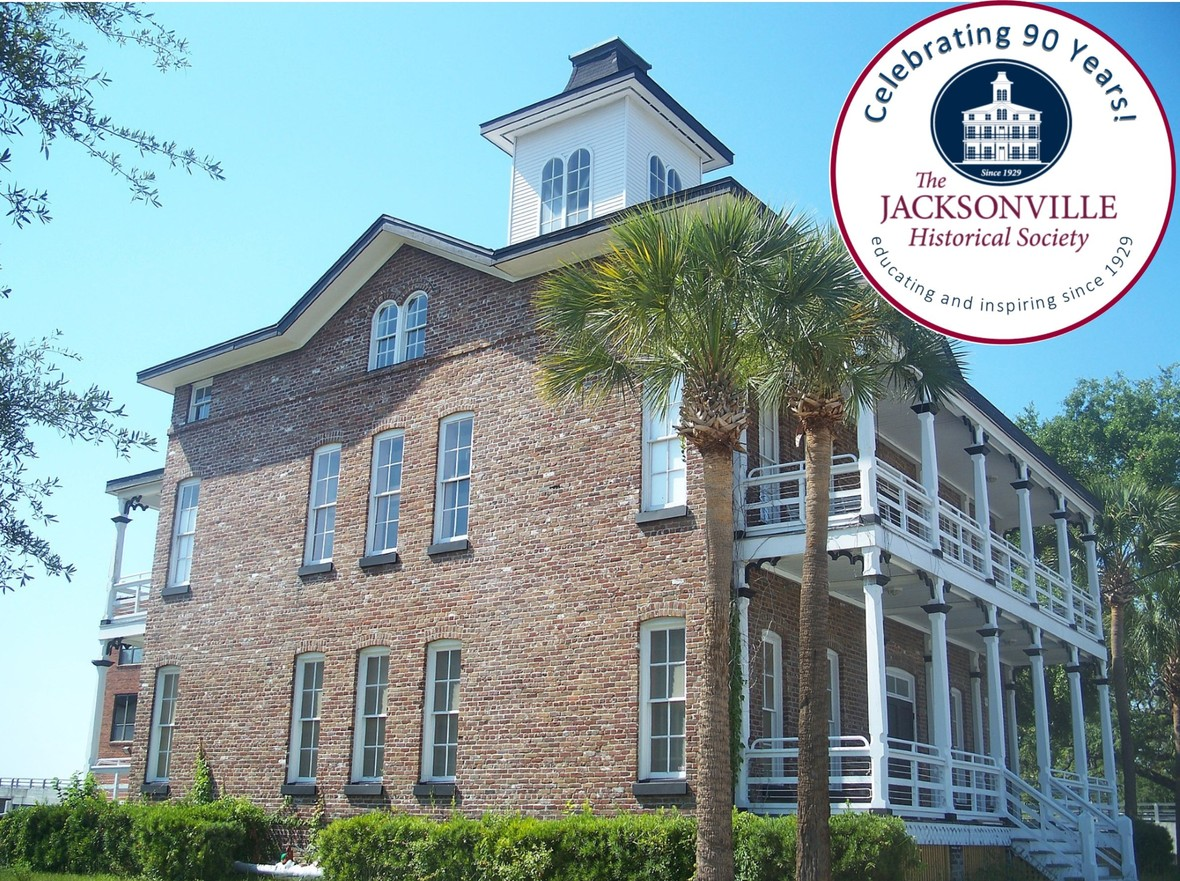On May 22 the Jacksonville Historical Society held its annual meeting at St. John’s Cathedral. We announced new officers, thanked our generous members and supporters, described projects underway, announced the 2019 “Endangered Buildings List,” and enjoyed refreshments while catching up with many friends. The capstone of the evening was the presentation by Dr. Wayne Wood, on Jacksonville’s era of grand hotels from 1869 to 1926.

We met at St. John’s Cathedral because of the unprecedented turnout. Well over 200 people registered in advance to attend, well beyond the capacity of our regular meeting place, Old St. Andrew’s Church. It seemed fitting to turn to another nearby historic church to accommodate our members and supporters. We are thankful to Dean Kate Moorehead and her terrific staff for their help and hospitality. Taliaferro Hall was full, but everyone had a good seat, with room to appreciate the memorabilia on display from the collections of Dr. Wood and Michael Fisher. The Cathedral was open at 5:00 for early arrivals to tour, and the Cathedral Gift Shop offered attendees a 20% discount coupon for future use.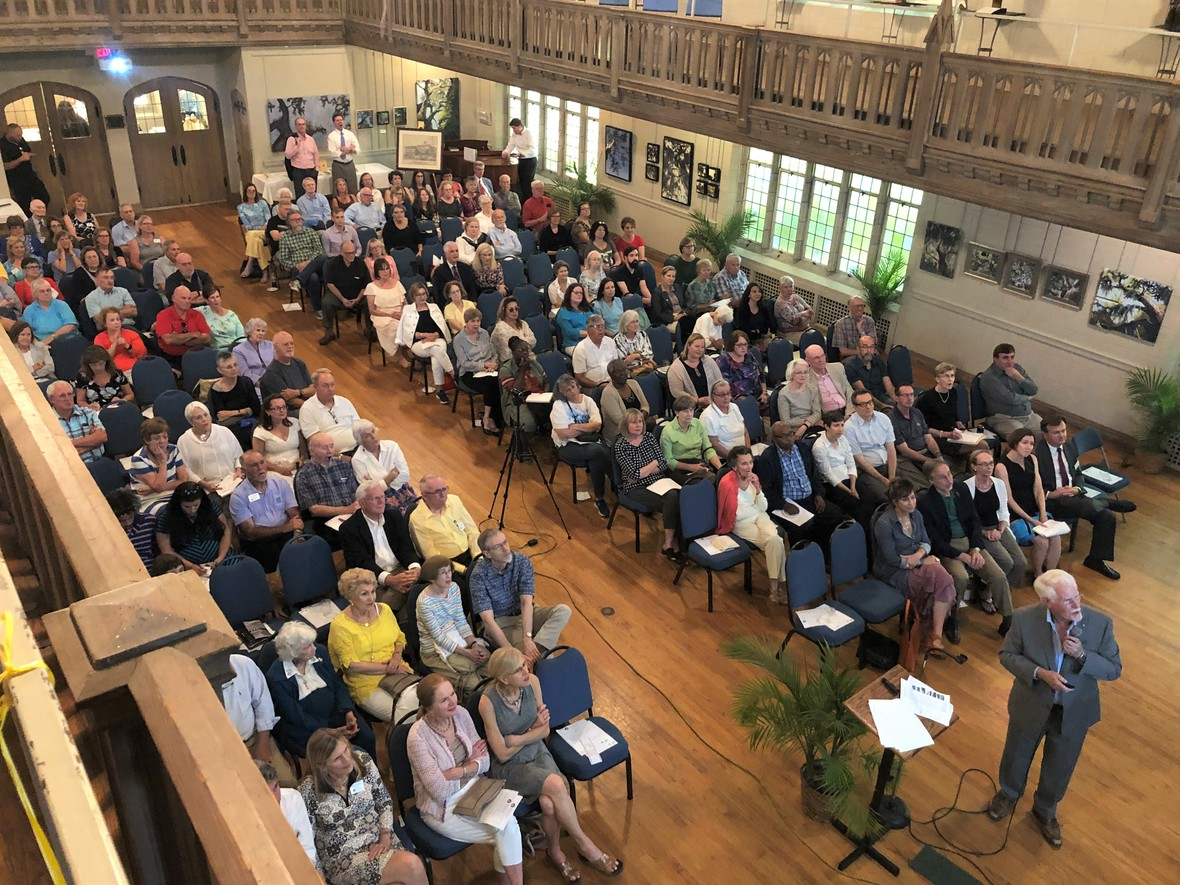/* styles */ The 2019 annual meeting was a great way to commemorate the anniversary of the founding of the Jacksonville Historical Society. After ninety years of service to Jacksonville, we stand on the shoulders of so many leaders and volunteers who came before us. Like them, we are motivated by our awareness that history matters. In Jacksonville, it matters especially to the people of a city that has more history than most places in Florida, but that is dispersed across a landscape bigger than any in this state, and most in the nation. Understanding that complicated past helps us be more certain of our place in the present, and points the way to our future. As stewards and educators, no one else in Jacksonville does what the JHS does. Back to the annual meeting: after the welcome and acknowledgements, JHS board member and past president Ed Booth, Jr. presented the 2019 list of Jacksonville’s most endangered buildings, developed by the board’s Historic Sites Committee. Booth announced that on Friday June 14th, the JHS will host a “Lunch & Learn” at Old St. Andrew’s where we will explore the list in greater detail, and discuss some of the issues and challenges in historic preservation. We will also consider some notable recent examples of “saves” of historic buildings – a happy context. Cost to attend will be \$15 for JHS members and \$20 for non-members. Lunch will be provided.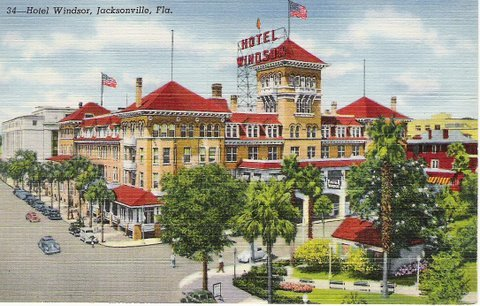/* styles */ Wayne Wood’s presentation was typically replete with fascinating images and photos, painstaking researched and narrated with insight. Dr. Wood’s service to local history and historic preservation is unmatched in Jacksonville, and the JHS is proud and fortunate to count him as a member, director and devoted supporter of our work. That work depends on the support of many, like you. Membership in the Jacksonville Historical Society is an investment in the future of this great, complicated city. Join, volunteer and donate. Discover the satisfaction that comes from learning and sharing about the people and events that make up our Jacksonville. Alan Bliss Executive Director
 table div table+table+table+table+table+table+table+table+table+table+table div table{width:100%;padding:0}table div table+table+table+table+table+table+table+table+table+table+table div table img{width:96.23%;padding:0;float:none}table div table+table+table+table+table+table+table+table+table+table+table div table td{width:100%;padding:0 1.88% 18px}/* styles */## Endangered Historic Properties Lunch & Learn: June 14

 table div table+table+table+table+table+table+table+table+table+table+table+table+table div table,table.module-12{width:32.08%;float:right;padding:0}table div table+table+table+table+table+table+table+table+table+table+table+table+table div table a{border:0 none;text-decoration:none}table div table+table+table+table+table+table+table+table+table+table+table+table+table div table img{width:100%!important;border:0 none;text-decoration:none}table div table+table+table+table+table+table+table+table+table+table+table+table+table div table td{width:100%;padding:0 0 20px 20px}/* styles */ Join us as we announce the 2019 Endangered Historic Properties List and discuss the state of historic preservation in Jacksonville. Experts in the field will describe recent successes, properties at risk, and best practices in our endeavors to preserve our historic treasurers. Make your reservation and order a Tidbits lunch at this link. WHEN: Friday, June 14, 11:30am WHERE: Old St. Andrews Church, 317 A. Philip Randolph Blvd. PARKING: Behind church.
 table div table+table+table+table+table+table+table+table+table+table+table+table+table+table+table div table{width:100%;padding:0}table div table+table+table+table+table+table+table+table+table+table+table+table+table+table+table div table img{width:96.23%;padding:0;float:none}table div table+table+table+table+table+table+table+table+table+table+table+table+table+table+table div table td{width:100%;padding:0 1.88% 18px}/* styles */## Deep Roots, Many Branches: The Blues in Northeast Florida - June 27

 table div table+table+table+table+table+table+table+table+table+table+table+table+table+table+table+table+table div table,table.module-16{width:32.08%;float:left;padding:0}table div table+table+table+table+table+table+table+table+table+table+table+table+table+table+table+table+table div table a{border:0 none;text-decoration:none}table div table+table+table+table+table+table+table+table+table+table+table+table+table+table+table+table+table div table img{width:100%!important;border:0 none;text-decoration:none}table div table+table+table+table+table+table+table+table+table+table+table+table+table+table+table+table+table div table td{width:100%;padding:0 20px 20px 0}/* styles */ We invite you to join us as Mitch Hemann, musician, singer-songwriter and JHS Archivist presents the origins and evolution of blues in Northeast Florida. From field hollers to the birth of Southern Rock, Jacksonville has played an important role in the formation of Blues music. Mitch Hemann will be your guide on a musical journey that twists and turns its way through more than 100 years of music history, tracing the origins and the evolution of this truly American art form. This unique presentation will feature a combination of speaking and live musical performance. Join us for this treat as Mitch unleashes his vocal chords and creativity to pay tribute to the legacy of blues in our region. This event is free for JHS members. A donation of \$10 is suggested for non-members. Become a JHS member here. WHERE: Old St. Andrews Church, 317 A. Philip Randolph Blvd. PARKING: Free event parking is available in the parking lot behind St. Andrews Church. JSO security will be on site. JHS Programs are possible through the generous support of Retina Associates, P.A., Fred H. Lambrou, Jr., M.D. and H. Alexander Leder, M.D.
 table div table+table+table+table+table+table+table+table+table+table+table+table+table+table+table+table+table+table+table div table{width:100%;padding:0}table div table+table+table+table+table+table+table+table+table+table+table+table+table+table+table+table+table+table+table div table img{width:96.23%;padding:0;float:none}table div table+table+table+table+table+table+table+table+table+table+table+table+table+table+table+table+table+table+table div table td{width:100%;padding:0 1.88% 18px}/* styles */## SAVE THE DATE: Salute to the Jax Red Caps - July 13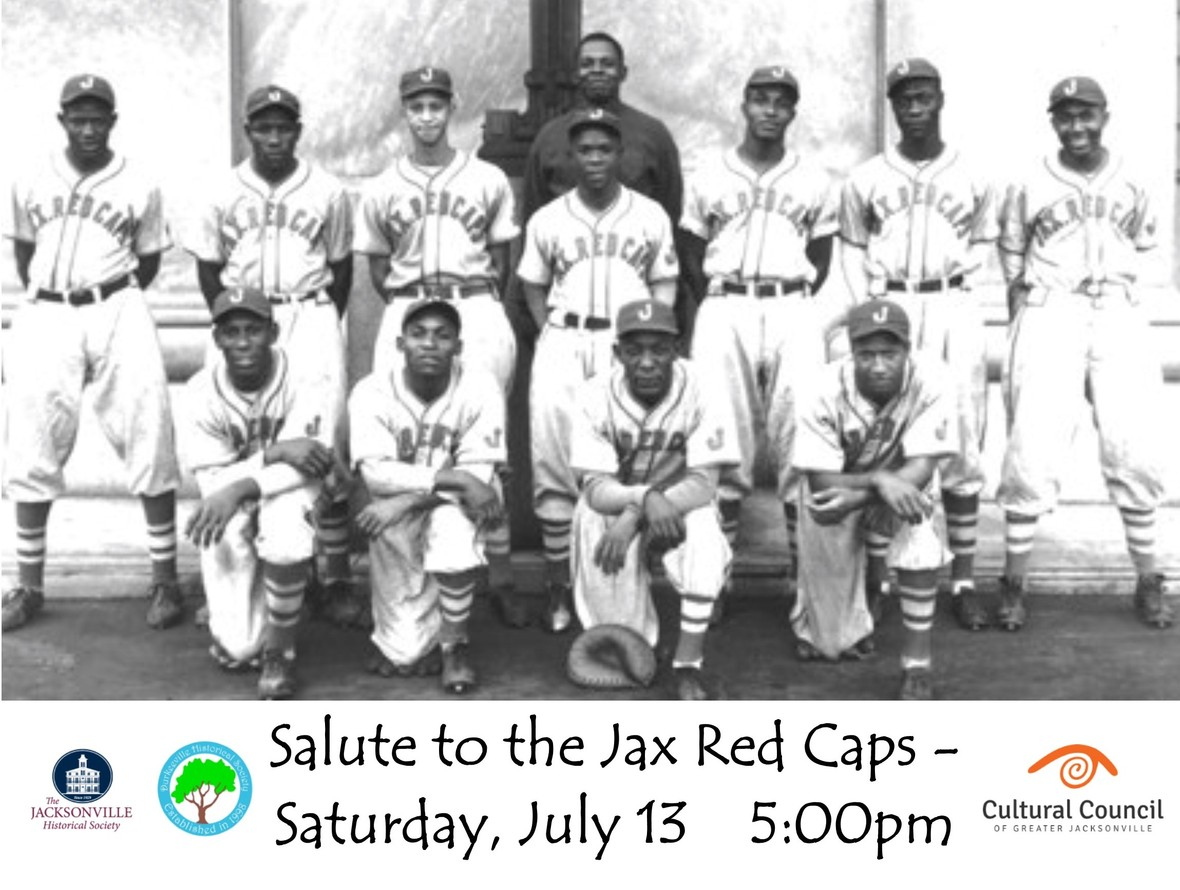SAVE THE DATE: Tickets available soon.
Wowza! Come on out for this triple play of summer entertainment.

5:00pm Salute to the Jax Red Caps presented by the Durkeeville Historical Society and the Jacksonville Historical Society.
6:00pm Walk to the ballpark with JHS and Durkeeville friends.
6:30pm Enjoy the Jax Jumbo Shrimp "Salute to the Red Caps" game in Section 110 with friends from JHS and Durkeeville Historical Society! Seats are located behind home plate on first base line.

 table div table+table+table+table+table+table+table+table+table+table+table+table+table+table+table+table+table+table+table+table+table+table div table{width:100%;padding:0}table div table+table+table+table+table+table+table+table+table+table+table+table+table+table+table+table+table+table+table+table+table+table div table img{width:96.23%;padding:0;float:none}table div table+table+table+table+table+table+table+table+table+table+table+table+table+table+table+table+table+table+table+table+table+table div table td{width:100%;padding:0 1.88% 18px}/* styles */## In Praise of Sanborn Maps

 /* styles */ Any seasoned researcher will tell you that one of the most prized resources you can have in your arsenal are Sanborn Maps. Weighing in at approximately 30 pounds, every volume of these massive books is a treasure trove of valuable information. That’s why we were so excited this month when we received a stack of these beauties to add to our map collection.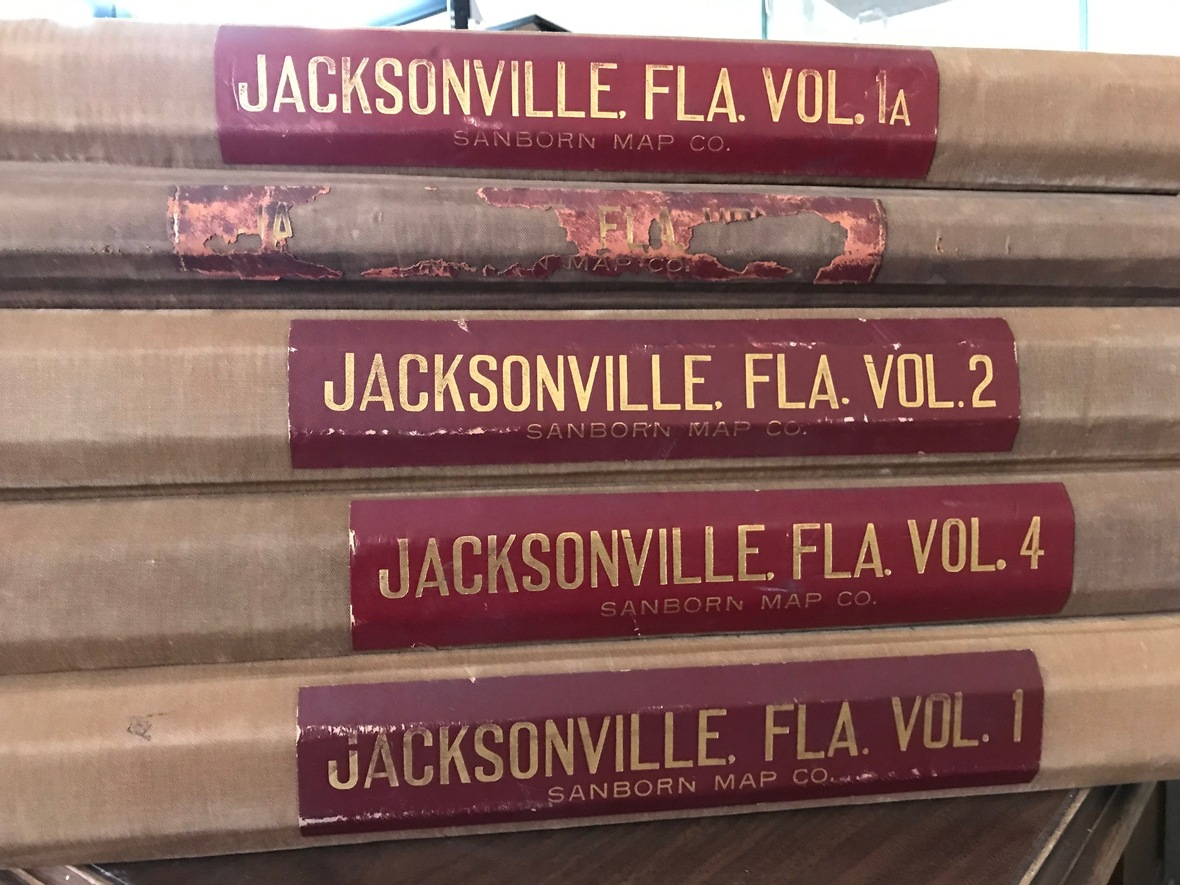Stack of early Sanborn Maps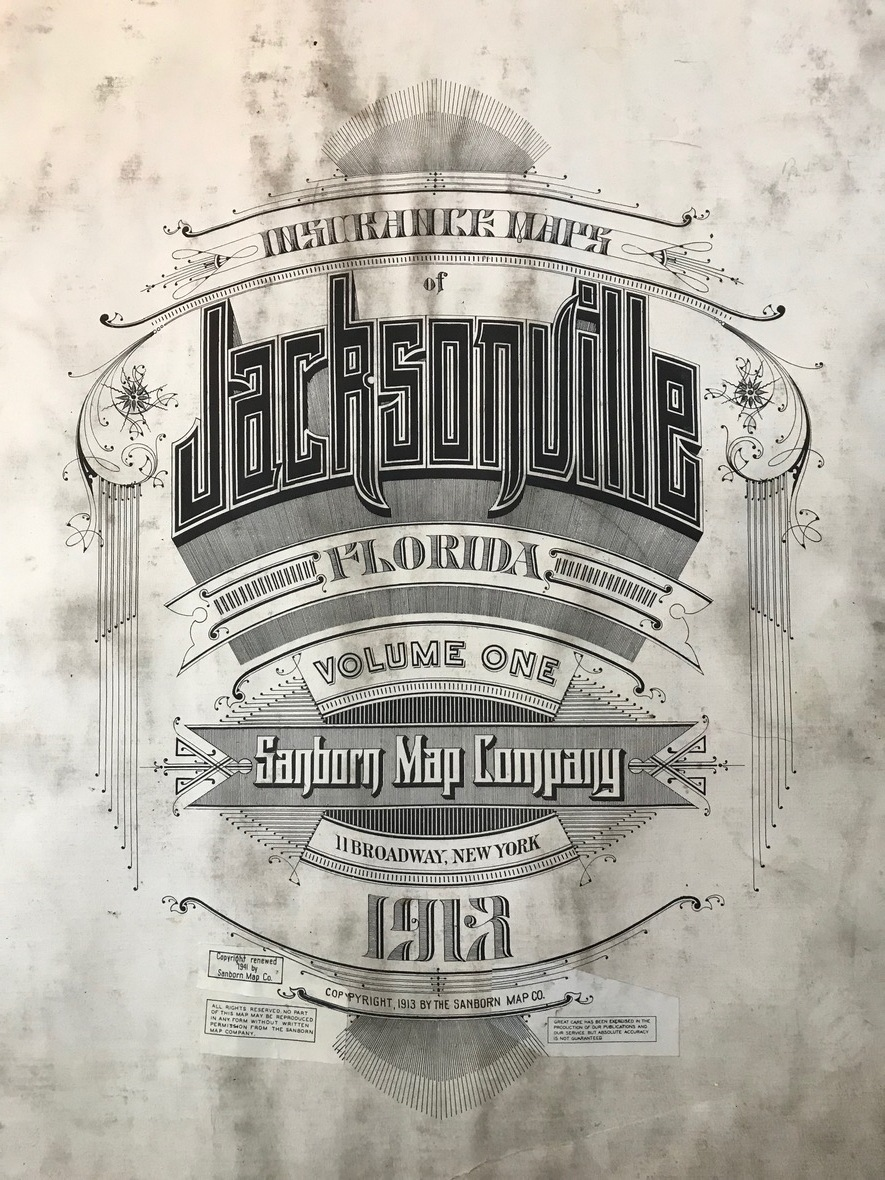Sanborn title page, 1913
 /* styles */ At the end of the Civil War, the Sanborn Map Publishing Company of New York City began printing complex and extremely detailed maps of cities across the nation. These maps were invaluable to insurance companies, who used them to estimate fire risk and determine appropriate insurance costs per building. These books were published all the way into the 1960s, and the largest collection of them are housed at the Library of Congress.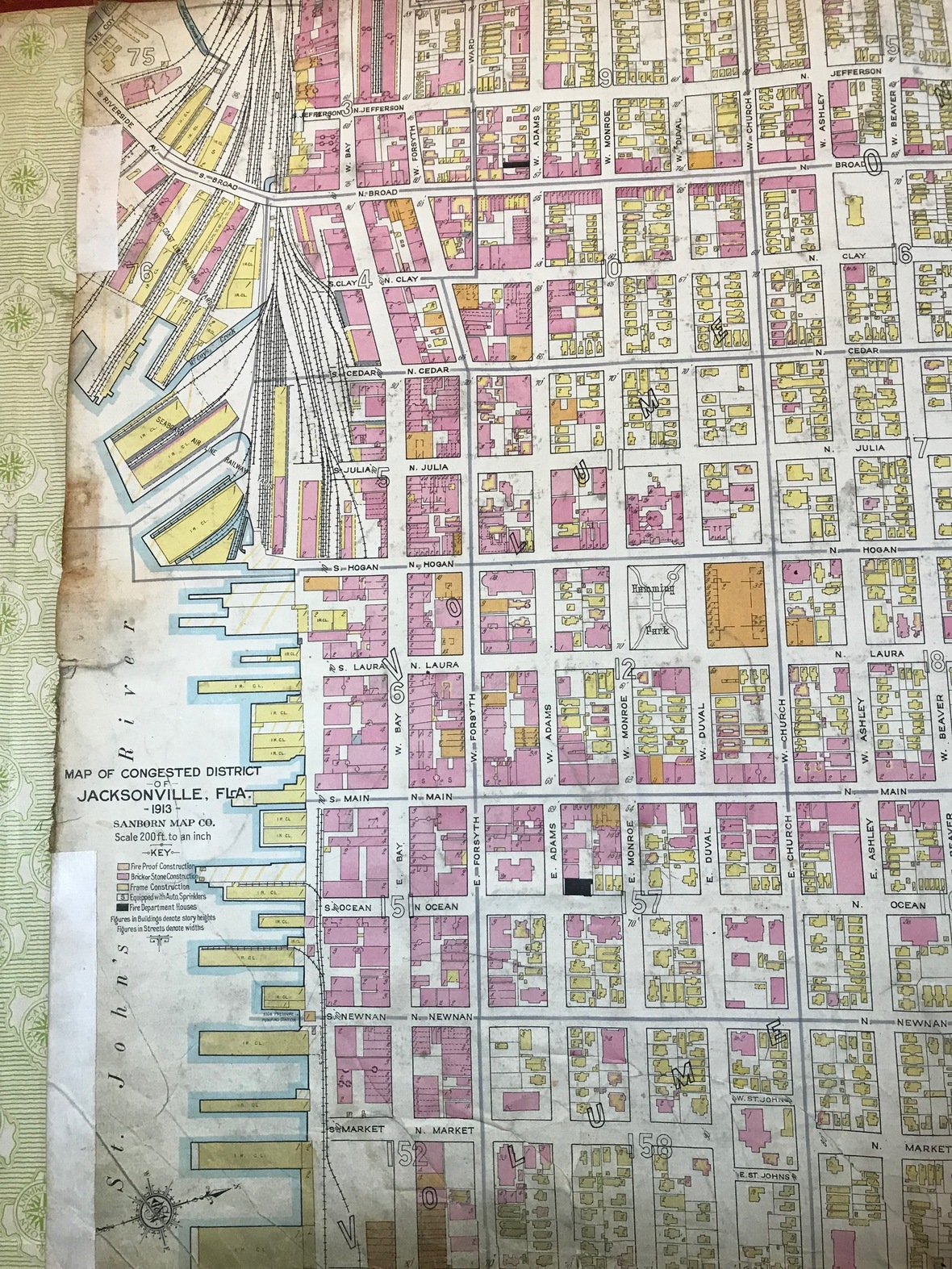A detailed view of early Jacksonville block by block
 /* styles */ Because of the many details these maps contain, they have wide appeal among researchers. From architectural historians and city planners to genealogists and preservationists, there’s something for everyone within these pages. Once you’ve decoded the key at the front of the book, you’ll be able to discover a wealth of information about these buildings and the streets on which they stood.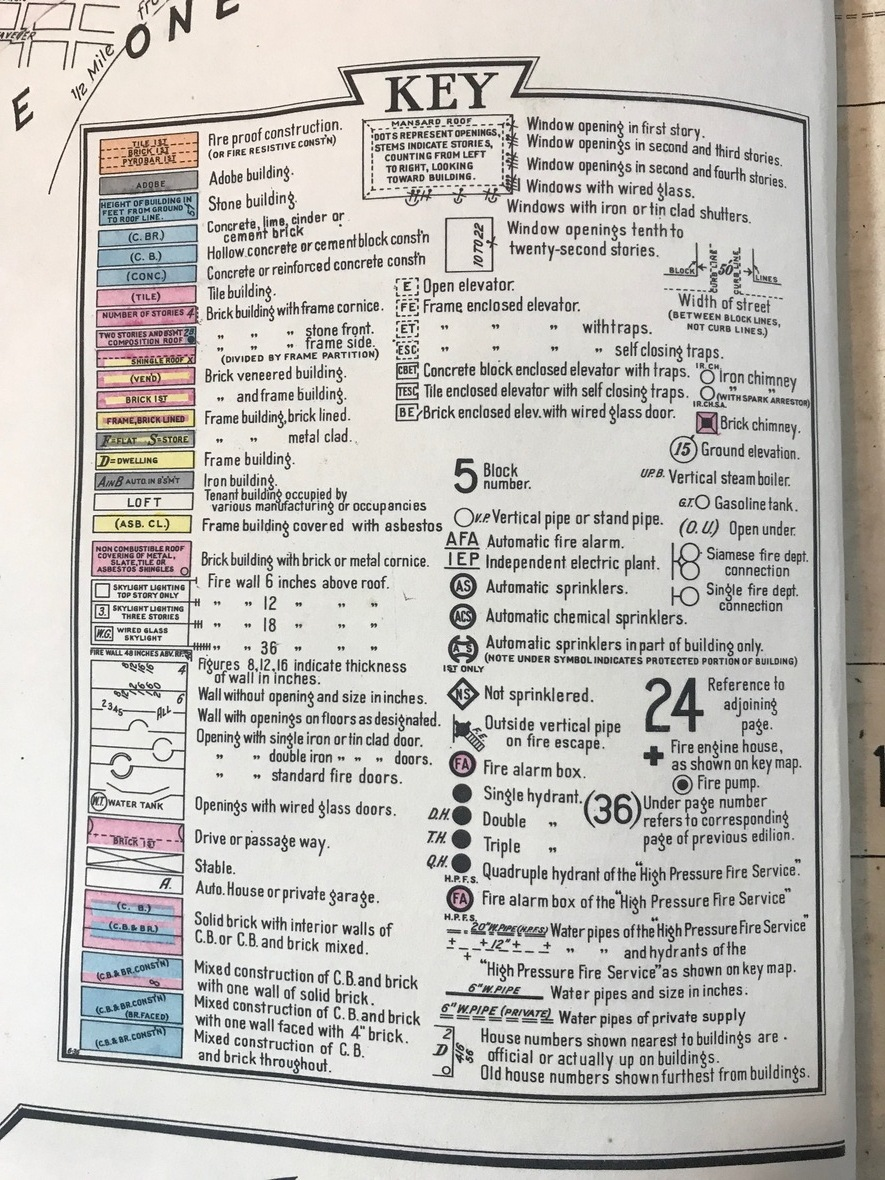The key at the front of the book is perhaps the most important page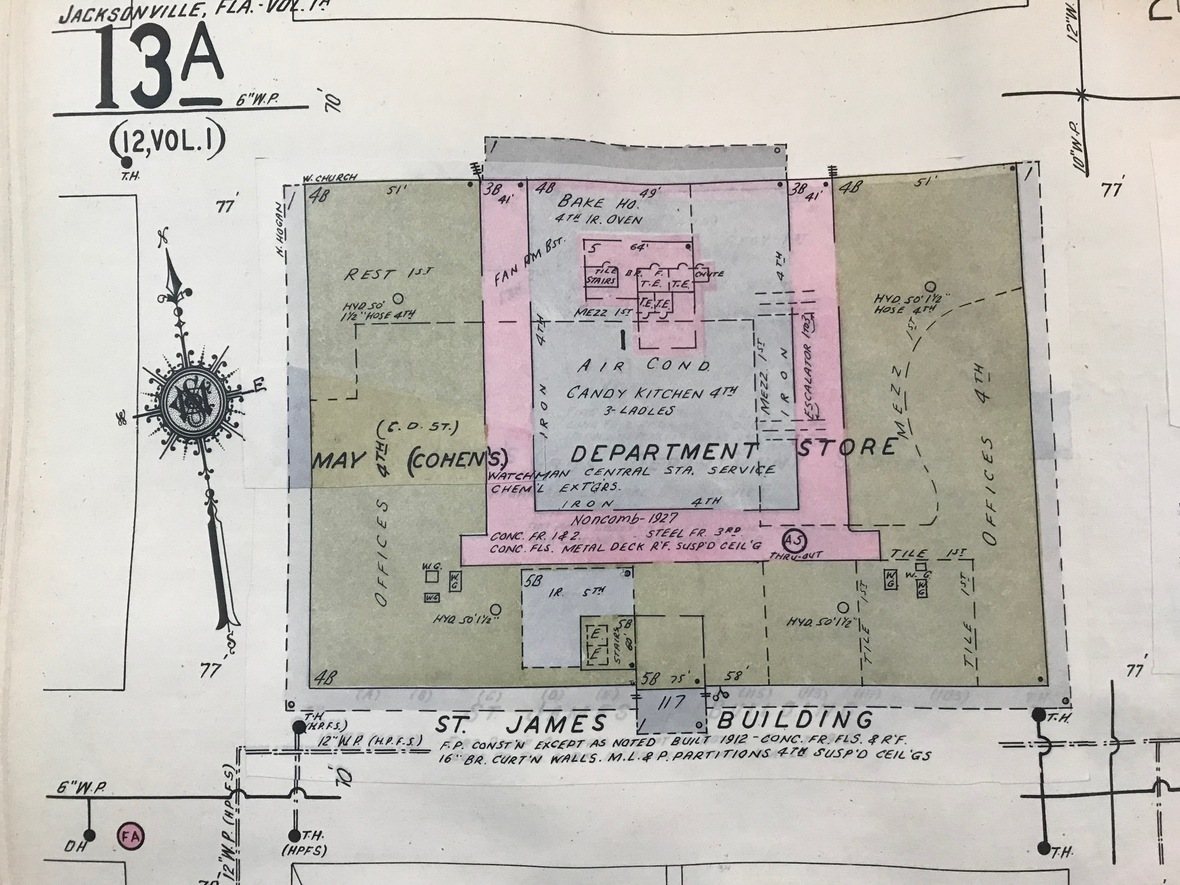Bird's eye view of May Cohen's Department Store on Duval Street
 /* styles */ These recent volumes have been generously donated to the Jacksonville Historical Society by J.F. Bryan IV, former president of Independent Insurance Group Inc. It’s with our heartfelt thanks to Mr. Bryan that these books are now available to the community to unlock the many secrets this city has to offer. by Mitch Hemann Archivist
 table div table+table+table+table+table+table+table+table+table+table+table+table+table+table+table+table+table+table+table+table+table+table+table+table+table+table+table+table+table+table+table+table+table div table{width:100%;padding:0}table div table+table+table+table+table+table+table+table+table+table+table+table+table+table+table+table+table+table+table+table+table+table+table+table+table+table+table+table+table+table+table+table+table div table img{width:96.23%;padding:0;float:none}table div table+table+table+table+table+table+table+table+table+table+table+table+table+table+table+table+table+table+table+table+table+table+table+table+table+table+table+table+table+table+table+table+table div table td{width:100%;padding:0 1.88% 18px}/* styles */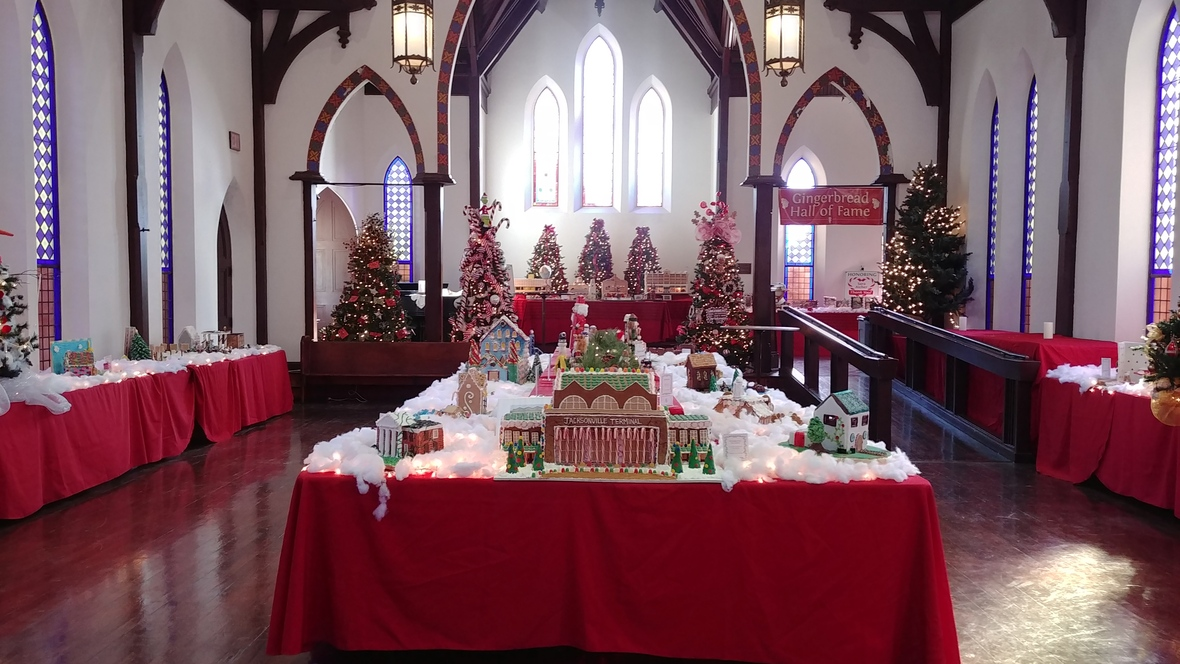## Gingerbread Builder Reservations Open

 /* styles */ Announcing the 2019 Gingerbread Builders' Sign Ups & Gingerbread Builders Workshop Reservations are being accepted for Builders for the 2019 Gingerbread Extravaganza. Test your creativity and skills in this cherished Jacksonville tradition. Build an 85% edible gingerbread creation that will be visited by thousands of gingerbread fans. Sign up here to reserve a spot.
 table div table+table+table+table+table+table+table+table+table+table+table+table+table+table+table+table+table+table+table+table+table+table+table+table+table+table+table+table+table+table+table+table+table+table+table+table+table+table div table{width:100%;padding:0}table div table+table+table+table+table+table+table+table+table+table+table+table+table+table+table+table+table+table+table+table+table+table+table+table+table+table+table+table+table+table+table+table+table+table+table+table+table+table div table img{width:96.23%;padding:0;float:none}table div table+table+table+table+table+table+table+table+table+table+table+table+table+table+table+table+table+table+table+table+table+table+table+table+table+table+table+table+table+table+table+table+table+table+table+table+table+table div table td{width:100%;padding:0 1.88% 18px}/* styles */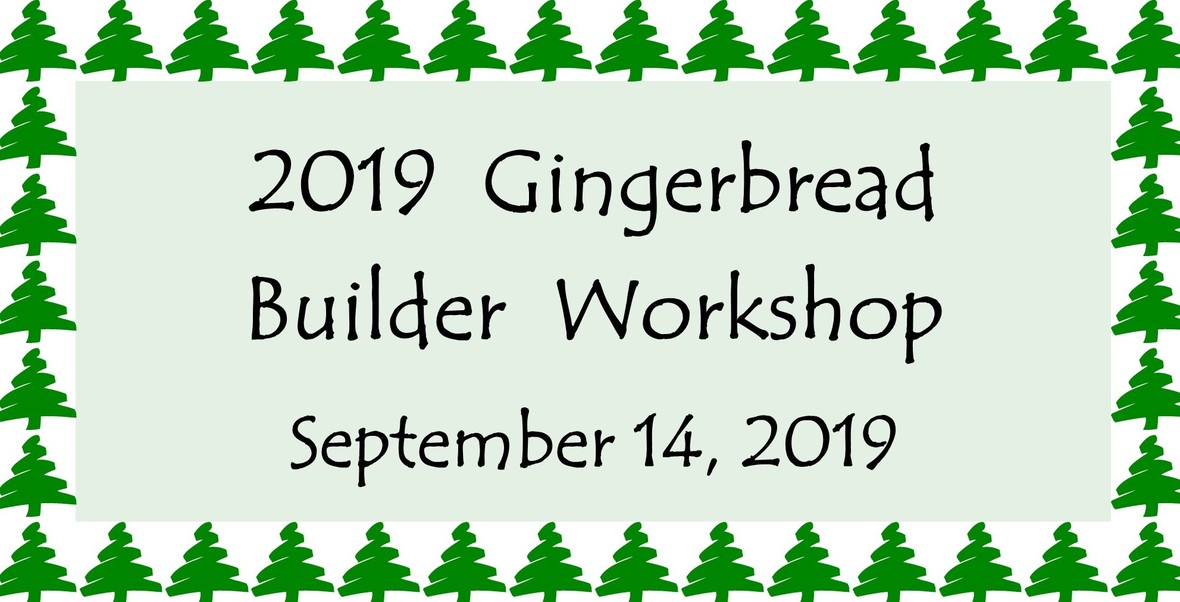## SAVE THE DATE: Gingerbread Builders' Workshop - September 14, 2019

 /* styles */ This is your chance. Get the advice we've all been waiting for ... Local experts and artists will instruct us on best practices for gingerbread construction on Saturday, September 14! This is a "DON'T MISS" event, if you are considering building for the 2019 Gingerbread Extravaganza. You will learn valuable tips and techniques in gingerbread construction and other edible building materials. This workshop will give you the combined confidence of Martha Stewart and Sir Isaac Newton and prepare you for a joyful building experience. Mark your calendar and reserve a spot.
 table div table+table+table+table+table+table+table+table+table+table+table+table+table+table+table+table+table+table+table+table+table+table+table+table+table+table+table+table+table+table+table+table+table+table+table+table+table+table+table+table+table+table+table div table{width:100%;padding:0}table div table+table+table+table+table+table+table+table+table+table+table+table+table+table+table+table+table+table+table+table+table+table+table+table+table+table+table+table+table+table+table+table+table+table+table+table+table+table+table+table+table+table+table div table img{width:96.23%;padding:0;float:none}table div table+table+table+table+table+table+table+table+table+table+table+table+table+table+table+table+table+table+table+table+table+table+table+table+table+table+table+table+table+table+table+table+table+table+table+table+table+table+table+table+table+table+table div table td{width:100%;padding:0 1.88% 18px}/* styles */## Help Us Raise the Profile of Old St. Andrew's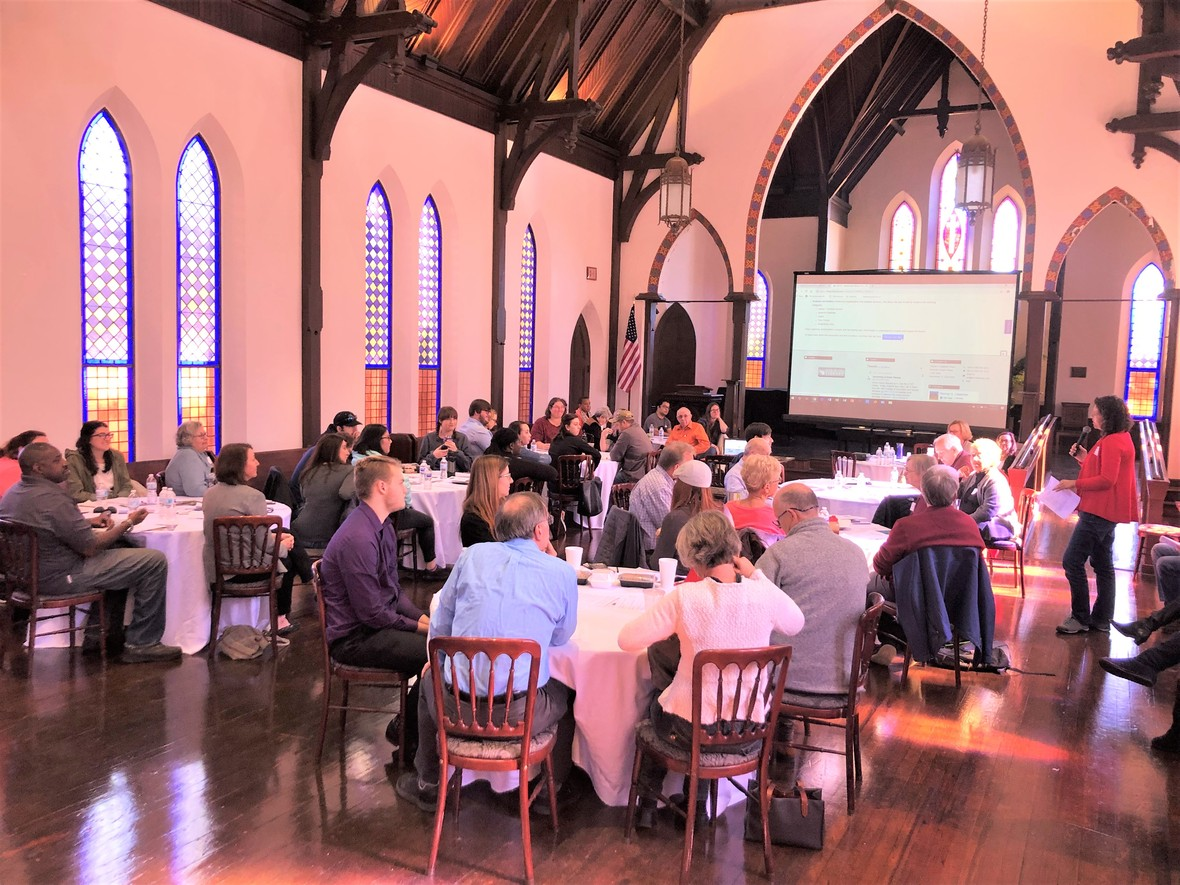"Charming", "vintage aesthetic" and "amazing" are just a few of the ways Old St. Andrews has been described by our customers. But competition continues unabated. You can help us raise the profile on this treasured facility through your social media accounts and online rating services!
1. Follow, Like, Share and Comment about Old St. Andrews Event Venue on your social media channels.
* Old St. Andrews Facebook: @oldstandrewsjax.
* Old St. Andrews Instagram: #oldstandrewsjax
2. Rate and comment on our Yelp page.
3. Write a Google review for Old St. Andrews.
4. Tell your friends about Old St. Andrews or invite them to one of the many events held within our impressive walls.

Your active and vocal representation of Old St. Andrews will allow it to continue to be a thriving and successful event venue in downtown Jacksonville!

Contact our Rental Coordinators soon to reserve space for your event!
Rob Jackson: 904-878-2241
Email: Events@JaxHistory.org

 table div table+table+table+table+table+table+table+table+table+table+table+table+table+table+table+table+table+table+table+table+table+table+table+table+table+table+table+table+table+table+table+table+table+table+table+table+table+table+table+table+table+table+table+table+table+table div table{width:100%;padding:0}table div table+table+table+table+table+table+table+table+table+table+table+table+table+table+table+table+table+table+table+table+table+table+table+table+table+table+table+table+table+table+table+table+table+table+table+table+table+table+table+table+table+table+table+table+table+table div table img{width:96.23%;padding:0;float:none}table div table+table+table+table+table+table+table+table+table+table+table+table+table+table+table+table+table+table+table+table+table+table+table+table+table+table+table+table+table+table+table+table+table+table+table+table+table+table+table+table+table+table+table+table+table+table div table td{width:100%;padding:0 1.88% 18px}/* styles */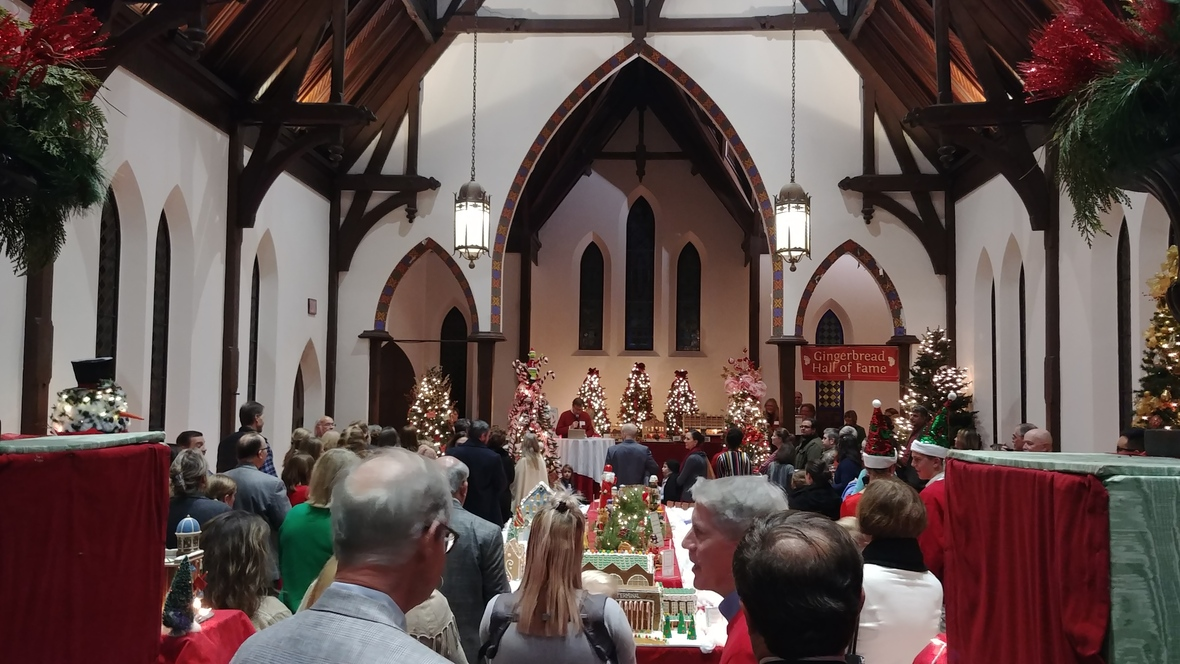## HOLIDAY PARTY RESERVATIONS at Old St. Andrews

 /* styles */ Old St. Andrews and the Gingerbread Extravaganza are available for evening holiday parties this December. Impress your staff by hosting your holiday party in the ambiance of Old St. Andrews. Our Rental Coordinators have catering resources ready to provide a turnkey event for you! For more information, contact our Rental Coordinators: Jade Stanley: 904-878-8122 Rob Jackson: 904-878-2241 Email: Events@JaxHistory.org
 table div table+table+table+table+table+table+table+table+table+table+table+table+table+table+table+table+table+table+table+table+table+table+table+table+table+table+table+table+table+table+table+table+table+table+table+table+table+table+table+table+table+table+table+table+table+table+table+table+table+table div table{width:100%;padding:0}table div table+table+table+table+table+table+table+table+table+table+table+table+table+table+table+table+table+table+table+table+table+table+table+table+table+table+table+table+table+table+table+table+table+table+table+table+table+table+table+table+table+table+table+table+table+table+table+table+table+table div table img{width:96.23%;padding:0;float:none}table div table+table+table+table+table+table+table+table+table+table+table+table+table+table+table+table+table+table+table+table+table+table+table+table+table+table+table+table+table+table+table+table+table+table+table+table+table+table+table+table+table+table+table+table+table+table+table+table+table+table div table td{width:100%;padding:0 1.88% 18px}/* styles */## Our Mission:

To educate and inspire the greater Jacksonville community to value its history, by fostering understanding of how the region's past shapes our present.

 table div table+table+table+table+table+table+table+table+table+table+table+table+table+table+table+table+table+table+table+table+table+table+table+table+table+table+table+table+table+table+table+table+table+table+table+table+table+table+table+table+table+table+table+table+table+table+table+table+table+table+table+table div table{width:100%;padding:0}table div table+table+table+table+table+table+table+table+table+table+table+table+table+table+table+table+table+table+table+table+table+table+table+table+table+table+table+table+table+table+table+table+table+table+table+table+table+table+table+table+table+table+table+table+table+table+table+table+table+table+table+table div table table{padding:0;float:left!important;width:52.604%!important}table div table+table+table+table+table+table+table+table+table+table+table+table+table+table+table+table+table+table+table+table+table+table+table+table+table+table+table+table+table+table+table+table+table+table+table+table+table+table+table+table+table+table+table+table+table+table+table+table+table+table+table+table div table td{padding-left:73px;padding-right:73px}table div table+table+table+table+table+table+table+table+table+table+table+table+table+table+table+table+table+table+table+table+table+table+table+table+table+table+table+table+table+table+table+table+table+table+table+table+table+table+table+table+table+table+table+table+table+table+table+table+table+table+table+table div table table td{padding-left:0;padding-right:20px}table div table+table+table+table+table+table+table+table+table+table+table+table+table+table+table+table+table+table+table+table+table+table+table+table+table+table+table+table+table+table+table+table+table+table+table+table+table+table+table+table+table+table+table+table+table+table+table+table+table+table+table+table div table table+table{float:left!important;width:47.396%!important}table div table+table+table+table+table+table+table+table+table+table+table+table+table+table+table+table+table+table+table+table+table+table+table+table+table+table+table+table+table+table+table+table+table+table+table+table+table+table+table+table+table+table+table+table+table+table+table+table+table+table+table+table div table table+table td{padding-left:0;padding-right:0}/* styles */

## Thank you for your support!

 table div table+table+table+table+table+table+table+table+table+table+table+table+table+table+table+table+table+table+table+table+table+table+table+table+table+table+table+table+table+table+table+table+table+table+table+table+table+table+table+table+table+table+table+table+table+table+table+table+table+table+table+table+table+table div table{width:100%;padding:0}table div table+table+table+table+table+table+table+table+table+table+table+table+table+table+table+table+table+table+table+table+table+table+table+table+table+table+table+table+table+table+table+table+table+table+table+table+table+table+table+table+table+table+table+table+table+table+table+table+table+table+table+table+table+table div table img{width:96.23%;padding:0;float:none}table div table+table+table+table+table+table+table+table+table+table+table+table+table+table+table+table+table+table+table+table+table+table+table+table+table+table+table+table+table+table+table+table+table+table+table+table+table+table+table+table+table+table+table+table+table+table+table+table+table+table+table+table+table+table div table td{width:100%;padding:0 1.88% 18px}/* styles */## Staff:

Alan Bliss, Ph.D. , Executive Director | Mitch Hemann, Archivist | Susan Prattos, Administrator | Imani Phillips, Archives & Office Assistant | Sherrard Ceglia, Archives Assistant | Anna Verney, Archives Assistant | David Woodard, Facilities Manager

## 2018-19 JHS Board

Michael Fackler, Esq., President | Frederick H. Kent III, Esq., Vice-President |
Jeffrey K. Graf, Treasurer | Charisse Thornton, Secretary | Pat Andrews, Immediate Past-President | Ed Booth, Esq. | David Chauncey, Esq. | Judge Gary Flower | Drew Haramis | Larry Kanter, M.D. | Doug Milne, Esq. | Harry Reagan | Skip Willbach | Maggie Means | Wayne W. Wood, O.D., Hon. AIA